# Texas Go Math Grade 6 Lesson 5.3 Answer Key Subtracting integers

Refer to our Texas Go Math Grade 6 Answer Key Pdf to score good marks in the exams. Test yourself by practicing the problems from Texas Go Math Grade 6 Lesson 5.3 Answer Key Subtracting integers.

## Texas Go Math Grade 6 Lesson 5.3 Answer Key Subtracting integers

Reflect

Question 1.
Communicate Mathematical Ideas Suppose you want to model the difference -4 – 7. Do you need to add zero pairs? If so, why? How many should you add? What is the difference?
You want to model:
– 4 – 7
First represent – 4 with 4 red counters.
You need to add some zero pairs because you need to remove 7 yellow counters
Since you need to remove 7 yellow counters, you should add 7 zero pairs.
When you remove yellow counters, there are 11 red counters left so the difference is:
– 4 – 7 = – 11
There are 4 red counters so you shouLd add 7 zero pairs in order to eliminate 7 yellow counters.
There are 11 red counters Left so the difference is – 11.

Question 2.
Communicate Mathematical Ideas Describe how to find 5 – (-8) on a number line. If you found the difference using counters, would you get the same result? Explain.
You want to find:
5 – (- 8)
Subtracting – 8 is equal to adding 8
so you find the sum
5 + 8
in order to find previous difference.
Graph number line with units.
Start at 5 and move 8 units right to represent adding 8.
The difference is 13 because it is 13 units right from 0 on the number line.

To find the difference using counters, start with 5 yellow counters to represent 5.
Add 8 zero pairs and remove 8 red counters to represent subtracting – 8
When you remove red counters, there are 13 yellow counters left so the difference is 13.

Subtracting – 8 is equal to adding its opposite 8 so find the sum 5 + 8 to find the difference 5 – (- 8).
The result is 13 because it is 13 units right from 0 on the number line.
You would get the same result if you used counters because there would be 13 yellow counters left.

Question 3.
What If? In Example 1, the temperature rose by 3 °C. Suppose it fell from -2 °C to -10 °C. Predict whether the change in temperature would be positive or negative. Then subtract to find the change.
The temperature was – 2 °C and fell to -10 °C so the change in temperature would be negative.
To find change in temperature, subtract first measured temperature from final temperature
– 10 – (- 2)
Subtracting – 2 is equal to adding its additive inverse 2 so you find sum:
– 10 + 2
First, subtract lesser absolute value from greater:
|- 10| – |2| = 8
Result would be 8 or – 8, depending on signs of given integers.
Use the sign of integer with greater absolute value to find change in temperature:
– 10 + 2 = -8

The change in temperature would be negative because the temperature fell.
The temperature fell 8 °C

Find each difference.

Question 4.
– 7 – 2 = ___________
Subtracting 2 is equal to adding its additive inverse – 2 so you can find sum:
– 7 + (- 2)
To calculate sum, find absolute values of integers:
|- 7| = 7 and |- 2| = 2
Then, sum that absolute values
7 + 2 = 9
Final result would be 9 or – 9, depending on sign of given integers (both positive then +, both negative then -).
Given integers are negative so final result is – 9.
Since finding difference – 7 – 2 is the same as finding sum – 7 + (- 2), the difference is – 9.

The difference is – 9.
To subtract 2, add its additive inverse – 2 and find sum using rules for adding integers.

Question 5.
– 1 – (-3) = ___________
Subtracting – 3 is equal to adding its additive inverse 3 so you can find sum:
– 1 + 3
To calculate sum, subtract lesser absolute value from greater:
|3| – |- 1| = 2
Result would be 2 or – 2, depending on signs of given integers
Use the sign of integer with greater absolute value to find the sum:
– 1 + 3 = 2
Since finding difference – 1 – (- 3) is the same as finding sum – 1 + 3, the difference is 2.

The difference is 2.
To subtract – 3, add its additive inverse 3 and find sum using rules for adding integers.

Question 6.
3 – 5 = ___________
Subtracting 5 is equal to adding its additive inverse – 5 so you can find sum:
3 – (- 5)
To calculate sum, subtract lesser absolute value from greater:
|- 5| – |3| = 2
Result would be 2 or – 2, depending on signs of given integers.
Use the sign of integer with greater absolute value to find the sum:
3 + (- 5) = – 2
Since finding difference 3 – 5 is the same as finding sum 3 + (- 5), the difference is – 2.

The difference is 2.
To subtract 5, add its additive inverse – 5 and find sum using rules for adding integers.

Question 7.
– 8 – (- 4) = ___________
Subtracting – 4 is equal to adding its additive inverse so you can find sum:
– 8 + 4
To calculate sum, subtract lesser absolute value from greater:
|- 8| – |4| = 4
Result would be 4 or – 4, depending on signs of given integers
Use the sign of integer with greater absolute value to find the sum:
– 8 + 4 = – 4
Since finding difference – 8 – (- 4) is the same as finding sum – 8 + 4, the difference is – 4.

The difference is – 4.
To subtract – 4, add its additive inverse 4 and find sum using rules for adding integers.

Explain how to find each difference using counters.

Question 1.
5 – 8 = _________
You want to find:
5 – 8
First, represent 5 with 5 yellow counters
Add 3 zero pairs because you need to remove 8 yellow counters in order to represent subtracting 8.
When you remove yellow counters, there are 3 red counters left so the difference is:
5 – 8 = -3

The difference is – 3.
Model 5 with 5 yellow counters, add 3 zero pairs and take away 8 yellow counters to represent subtracting 8.

Question 2.
– 5 – (-3) = _________
You want to find:
– 5 – (- 3)
First, represent – 5 with 5 red counters.
You need to remove 3 red counters in order to represent subtracting – 3.
When you remove 3 red counters1 there are 2 red counters left so the difference ¡S:
– 5 – (- 3) = – 2

The difference is – 2.
Model – 5 with 5 red counters and remove 3 red counters to represent subtracting – 3.

Use a number line to find each difference.

Question 3.
– 4 – 5 = – 4 + _____ = _____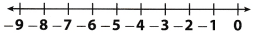You want to find:
– 4 – 5
Subtracting 5 is equal to adding – 5
so you find the sum
– 4 + (- 5)
in order to find previous difference.
Graph number line with units.
Start at – 4 and move 5 units left to represent adding – 5.
Read the difference on the number tine.

Number line: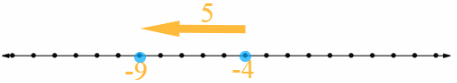The difference is – 9 because it is 9 units left from 0 on the number line.

Question 4.
1 – 4 = 1 + _____ = _____You want to find:
1 – 4
Subtracting 4 is equal to adding – 4
so you find the sum
1 + (- 4)
in order to find previous difference.
Graph number line with units.
Start at 1 and move 4 units left to represent adding – 4.
Read the difference on the number tine.

Number line: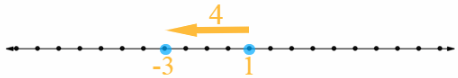The difference is – 3 because it is 3 units left from 0 on the number line.

Solve.

Question 5.
8 – 11 = ______________
Subtracting 11 is equal to adding its additive inverse – 11 so you can find sum:
8 + (- 11)
To calculate sum, subtract lesser absolute value from greater:
|- 11| – |8| = 3
Result would be 3 or – 3, depending on signs of given integer&
Use the sign of integer with greater absolute value to find the sum:
8 + (-11) = – 3
Since finding difference 8 – 11 is the same as finding sum 8 + (- 11), the difference is – 3.

The difference is – 3.
To subtract 11, add its additive inverse – 11 and find sum using rules for adding integers.

Question 6.
– 3 – (-5) = ______________
Subtracting – 5 is equal to adding its additive inverse 5 so you can find sum:
– 3 + 5
To calculate sum, subtract lesser absolute value from greater:
|5| – |- 3| = 2
Result would be 2 or – 2, depending on signs of given integers.
Use the sign of integer with greater absolute value to find the sum:
– 3 + 5 = 2
Since finding difference – 3 – (- 5) is the same as finding sum – 3 + 5, the difference is 2.

The difference is 2
To subtract – 5, add its additive inverse 5 and find sum using rules for adding integers.

Question 7.
15 – 21 = ______________
Subtracting 21 is equal to adding its additive inverse – 21 so you can find sum:
15 + (- 21)
To calculate sum, subtract lesser absolute value from greater:
|- 21| – |15| = 6
Result would be 6 or – 6, depending on signs of given integers.
Use the sign of integer with greater absolute value to find the sum:
15 + (- 21) = – 6
Since finding difference 15 – 21 is the same as finding sum 15 + (- 21), the difference is – 6.

The difference is – 6.
To subtract 21 add its additive inverse – 21 and find sum using rules for adding integers.

Question 8.
– 17 – 1 = ______________
Subtracting 1 is equal to adding its additive inverse – 1 so you can find sum:
– 17 + (- 1)
To calculate sum, find absolute values of integers:
|- 17| = 17 and |- 1| = 1
Then, sum that absolute values
17 + 1 = 18
Final result would be 18 or -18, depending on sign of given integers (both positive then +, both negative then -).
Given integers are negative so final result is – 18.
Since finding difference – 17 – 1 is the same as finding sum -17 + (- 1), the difference is -18.

The difference is – 18.
To subtract 1, add its additive inverse – 1 and find sum using rules for adding integers.

Question 9.
0 – (-5) = ______________
Subtracting – 5 is equal to adding its additive inverse 5 so you can find sum:
0 + 5
Sum of 0 and any integer is equal to that integer so the sum is 5.
Since finding difference 0 – (- 5) is the same as finding sum 0 + 5, the difference is 5.

The difference is 5.
To subtract – 5, add its additive inverse 5 and find sum using rules for adding integers.

Question 10.
1 – (-18) = ______________
Subtracting – 18 is equal to adding its additive inverse 18 so you can find sum:
1 + 18
The sum is:
1 + 18 = 19
Since finding difference
1 – ( – 18)
is the same as finding sum
1 + 18
the difference is 19.
To subtract – 18, add its additive inverse 18 and find sum using rules for adding integers.

Question 11.
15 – 1 = ______________
Subtracting 1 is equal to adding its additive inverse – 1 so you can find sum:
15 + (- 1)
To calculate sum, subtract lesser absolute value from greater;
|15| – |- 1| = 14
Result would be 14 or – 14, depending on signs of given integers.
Use the sign of integer with greater absolute value to find the sum:
15 + (- 1) = 14
Since finding difference 15 – 1 is the same as finding sum 15 + (- 1), the difference is 14.

The difference is 14.
To subtract 1, add its additive inverse – 1 and find sum using rules for adding integers.

Question 12.
– 3 – (-45) = ______________
Subtracting – 45 is equal to adding its additive inverse 45 so you can find sum:
– 3 + 45
To calculate sum, subtract lesser absolute value from greater:
|45| – |- 3| = 42
Result would be 42 or – 42, depending on signs of given integers.
Use the sign of integer with greater absolute value to find the sum:
– 3 + 45 = 42
Since finding difference – 3 – (- 45) is the same as finding sum – 3 + 45, the difference is 42.

The difference is 42.
To subtract – 45, add its additive inverse 45 and find sum using rules for adding integers.

Question 13.
19 – (-19) = ______________
Subtracting -19 is equal to adding its additive inverse 19 so you can find sum:
19 + 19
The sum is
19 + 19 = 38
Since findIng difference 19 – (- 19) b the same as finding sum 19 + 19. the difference is 38.

The difference is 38.
To subtract – 19, add its additive inverse 19 and find sum using rules for adding integers.

Question 14.
– 87 – (- 87) = ______________
Subtracting – 87 is equal to adding its additive inverse 87 so you can find sum:
– 87 + 87
Sum of integer and its additive inverse is 0
so the result is:
– 87 + 87 = 0
Since finding difference – 87 – (- 87) is the same as finding sum – 87 + 87, the difference is 0.

The difference is 0.
To subtract – 87, add its additive inverse 87 and find sum using rules for adding integers.

Essential Question Check-In

Question 15.
How do you subtract an integer from another integer without using a number line or counters? Give an example.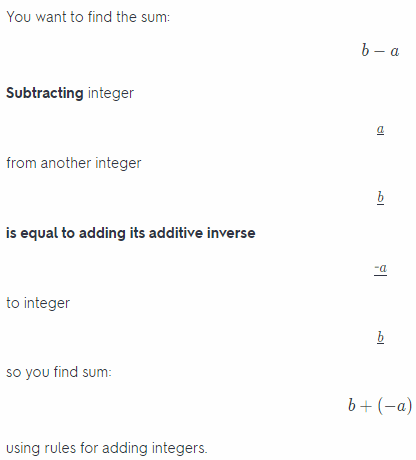For example, the difference:
15 – 6
is equal to the sum:
15 + (- 6)
where – 6 is additive inverse of 6.
To calculate sum, subtract lesser absolute value from greater:
|15| – |- 6| = 9
Result would be 9 or – 9, depending on signs of given integers.
Use the sign of integer with greater absolute value to find the sum:
15 + (- 6) = 9
Since finding difference 15 – 6 is the same as finding sum 15 + (- 6), the difference is 9.

Subtracting integer a front another integer b is equal to adding its additive inverse – a to integer b so you find sum:
b + (- a)
For example, the difference 15 – 6 is equal to the sum 15 + (- 6) where – 6 is additive inverse of 6.

Question 16.
Theo had a balance of – $4 in his savings account. After making a deposit, he has$25 in his account. What is the overall change to his account?
The overall change to Theo’s account is the difference between current balance and balance before making a deposit:
25 – (- 4)
Subtracting – 4 is equal to adding its additive inverse 4 so you can find sum:
25 + 4
The sum is:
25 + 4 = 29
Since finding difference 25 – (- 4) is the same as finding sum 25 + 4, the difference is 29.

The overall change to Theo’s account is 29.
Find the difference 25 – (- 4) to represent change to his account.

Question 17.
As shown, Suzi starts her hike at an elevation below sea level. When she reaches the end of the hike, she is still below sea level at – 127 feet. What was the change in elevation from the beginning of Suzi’s hike to the end of the hike?The change in elevation from the beginning to the end of Suzi’s hike ¡s the difference:
– 127 – (- 225)
Subtracting – 225 is equal to adding its additive inverse 225 so you can find sum:
– 127 + 225
To calculate sum, subtract lesser absolute value from greater:
|225| – |- 127| = 98
Result would be 98 or – 98, depending on signs of given integers.
Use the sign of integer with greater absolute value to find the sum:
– 127 + 225 = 98
Since finding difference – 127 – (- 225) ¡s the same as finding sum – 127 + 225, the difference is 98.

The change in elevation was 98 feet
Find the difference – 127 – (-225) to represent the change in elevation.

Question 18.
The record high January temperature in Austin, Texas, is 90 °F. The record low January temperature is – 2 °F. Find the difference between the high and low temperatures.
Find the difference:
90 – (- 2)
Subtracting – 2 is equal to adding its additive inverse 2 so you can find sum:
90 + 2
The sum is:
90 + 2 = 92
Since finding difference 90 – (- 2) is the same as finding sum 90 + 2, the difference is 92.

The difference between high and low temperatures in Austin is 92 °F.
Find the difference 90 – (-2) to represent that difference.

Question 19.
Cheyenne is playing a board game. Her score was – 275 at the start of her turn, and at the end of her turn her score was – 425. What was the change in Cheyenne’s score from the start of her turn to the end of her turn?
Find the difference
– 425 – (- 275)
to represent the change in Cheyenne’s score.
Subtracting – 275 is equal to adding its additive inverse 275 so you can find sum:
– 425 + 275
To calculate sum, subtract lesser absolute value from greater:
|- 425| – |275| = 150
Result would be 150 or – 150, depending on signs of given integers.
Use the sign of integer with greater absolute value to find the sum:
– 425 + 275 = – 150
Since finding difference – 425 – (-275) is the same as finding sum – 425 + 275, the difference is – 150.

The change in Cheyenne’s score was – 150.
Find the difference – 425 – (-275) to represent the change in her score.

Question 20.
A scientist conducts three experiments in which she records the temperature of some gases that are being heated. The table shows the initial temperature and the final temperature for each gas.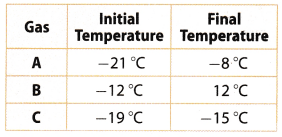a. Write a difference of Integers to find the overall temperature change for each gas.
Gas A: _________________
The temperature change for gas A is the difference:
– 8 – (- 21)
Subtracting – 21 is equal to adding its additive inverse 21 so you can find sum:
– 8 + 21
To calculate sum, subtract lesser absolute value from greater:
|21| – |- 8| = 13
Result would be 13 or – 13, depending on signs of given integers.
Use the sign of integer with greater absolute value to find the sum:
– 8 + 21 = 13
Since finding difference – 8 – (- 21) is the same as finding sum – 8 + 21, the difference is 13.

Gas B: _________________
The temperature change for gas B is the difference:
12 – (-12)
Subtracting -12 is equal to adding its additive inverse 12 so you can find sum:
12 + 12
The sum is:
12 + 12 = 24
Since finding difference 12 – (-12) is the same as finding sum 12 + 12, the difference is 24.

Gas C: _________________
The temperature change for gas C is the difference:
– 15 – (- 19)
Subtracting – 19 is equal to adding its additive inverse 19 so you can find sum:
– 15 + 19
To calculate sum, subtract lesser absolute value from greater:
|19| – |- 15| = 4
Result would be 4 or – 4, depending on signs of given integers.
Use the sign of integer with greater absolute value to find the sum:
– 15 + 19 = 4
Since finding difference -15 – (-19) is the same as finding sum -15 + 19, the difference is 4.

b. What If? Suppose the scientist performs an experiment in which she cools the three gases. Will the changes in temperature be positive or negative for this experiment? Why?
If the scientist conducts the experiment in which she cools these gases, temperature change will be negative because temperature of each gas will decrease.

Question 21.
Analyze Relationships For two months, Nell feeds her cat Diet Chow brand cat food. Then for the next two months, she feeds her cat Kitty Diet brand cat food. The table shows the cat’s change in weight over 4 months.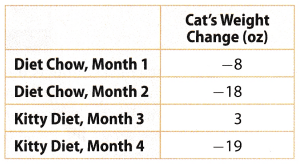Which brand of cat food resulted in the greatest weight loss for Nell’s cat? Explain.
Calculate cat’s change in weight over first two months:
– 8 + (- 18)
To calculate sum, find absolute values of integers:
|- 8| = 8 and |- 18| = 18
Then, sum that absolute values
8 + 18 = 26
Result would be 26 or – 26, depending on sign of given integers (both positive then +, both negative then -).
Given integers are negative so result is – 26.

Calculate cat’s change in weight over third and forth month:
3 + (- 19)
To calculate sum, subtract lesser absolute value from greater:
|- 19| – |3| = 16
Result would be 16 or – 16, depending on signs of given integers.
Use the sign of integer with greater absolute value to find the sum:
3 + (- 19) = – 16
First brand, Diet Chow, resulted in greater weight loss because Nell’s cat lost 26oz while eating that food.

Nell’s cat lost 26oz while eating Diet Chow cat food and cat lost l6oz while eating Kitty Diet cat food so the first brand, Diet Chow, resulted in greater weight loss.

H.O.T. Focus On Higher Order Thinking

Question 22.
Represent Real-World Problems Write and solve a word problem that can be modeled by the difference – 4 – 10.
Mary has 10 points after first play and her total score after second play is – 4 points. Find Mary’s score on her second play.
Subtract Mary’s score after first play from her total score to find score in second play:
– 4 – 10
Subtracting 10 is equal to adding its additive inverse – 10 so you can find sum:
– 4 + (- 10)
To calculate sum, find absolute values of integers:
|- 4| = 4 and |- 10| = 10
Then, sum that absolute values
4 + 10 = 14
Result would be 14 or – 14, depending on sign of given integers (both positive then +, both negative then -).
Given integers are negative so the result is – 14.
Since finding difference – 4 – 10 is the same as finding sum – 4 + (- 10), the difference is – 14.

Mary has 10 points after first play and her total score after second play is – 4 points. Find Mary’s score on her second play.
Mary’s score on her second play is – 14 points.
To subtract 10, add its additive inverse – 10 and find sum using rules for adding integers.

Question 23.
Explain the Error When Tom found the difference – 11 – (- 4), he got – 15. What might Tom have done wrong?
The difference is:
– 11 – (- 4)
Tom found that the result is – 15.
Subtracting – 4 is equal to adding its additive inverse 4 so you can find sum:
– 11 + 4
To calculate the sum, subtract lesser absolute value from greater
|- 11| – |4| = 7
Final result would be 7 or – 7, depending on signs of given integers.
Use the sign of integer with greater absolute value to find the sum:
-11 + 4 = – 7
Since finding difference – 11 – (- 4) is the same as finding sum – 11 + 4, the difference is – 7.

The difference is – 7.
To subtract – 4, add its additive inverse 4 and find sum using rules for adding integers.

Question 24.
Draw Conclusions When you subtract one negative integer from another, will your answer be greater than or less than the integer you started with? Explain your reasoning and give an example.
The result of subtracting one negative integer from another
a – b
would be greater than the integer you started with, a.
Subtracting negative integer b is equal to adding its additive inverse, – b, which is positive integer, so you get sum
a + (- b)
Use the number line:
Start at a and move to the right on the number line because you add positive integer, – b.
The result is right from a on the number line so it is greater than a.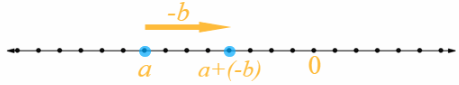For example, find the difference:
– 7 – (- 5)
Subtracting -5 is equal to adding its additive inverse 5 so you can find sum:
– 7 + 5
Graph number line with units.
Start at – 7 and move 5 units right to represent adding 5.
The sum is – 2 because it is 2 units left from 0 on the number line.
Since finding difference – 7 – (- 5) is the same as finding sum – 7 + 5, the difference is – 2.
Integer – 2 is greater than the integer you started with, – 7 because it is right from – 7 on the number line.

The result of subtracting one negative integer from another would be greater than the integer you started with.
For example, – 7 – (-5) = – 2.
Integer – 2 is greater than integer you started with, – 7, because it is right from – 7 on the number line.

Question 25.
Look for a Pattern Find the next three terms in the pattern 9, 4, – 1, – 6, – 11 Then describe the pattern.
The pattern is:
9, 4, – 1, – 6, – 11, …….
Next three terms in this pattern are
– 16, – 21, – 26
Every next term is
the difference of previous term and integer 5
For example:
9 – 5 = 4
Subtracting 5 is equal to adding its additive inverse – 5 so you can find sum:
9 + (- 5)
To calculate sum, subtract lesser absolute value from greater:
|9| – |- 5| = 4
Result would be 4 or – 4, depending on signs of given integers.
Use the sign of integer with greater absolute value to find the sum:
9 + (- 5) = 4
Since finding difference 9 – 5 is the same as finding sum 9 + (- 5), the difference is 4.

You can find next terms in a similar way:
4 – 5 = – 1
– 1 – 5 = – 6
– 6 – 5 = – 11
– 11 – 5 = – 16
– 16 – 5 = – 21
– 21 – 5 = – 26

Next three terms in this pattern are – 16, – 21, – 26.
Every next term is the difference of previous term and integer 5.

Scroll to Top